Courses

# Extra Questions- Cubes and Cube Roots Class 8 Notes | EduRev

## Class 8 Mathematics by Full Circle

Created by: Full Circle

## Class 8 : Extra Questions- Cubes and Cube Roots Class 8 Notes | EduRev

The document Extra Questions- Cubes and Cube Roots Class 8 Notes | EduRev is a part of the Class 8 Course Class 8 Mathematics by Full Circle.
All you need of Class 8 at this link: Class 8

Question 1: Show that –1728 is a perfect cube. Also, find the number whose cube is –1728.

Solution: We have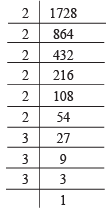i.e. 1728 = 2 * 2 * 2 * 2 * 2 * 2 * 3 * 3 * 3

⇒ 1728 = 23 * 23 * 33

⇒ 1728 = (2 * 2 * 3)3

⇒ ∛1728 = 2 * 2 * 3 = 12

Since 1728 is a perfect cube.

∴ –1728 is also a perfect cube.

Also ∛-1728 = –12

⇒ –1728 is a perfect cube of –12.

Question 2: Is 216 a perfect cube? What is the number whose cube is 216?

Solution: We have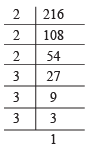i.e. Resolving 216 into prime factors we have:

216 = 2 * 2 * 2 * 3 * 3 * 3

i.e. 216 can be resolved in to such prime factor which can be grouped into triple of equal of factor and no factor is left over.

∴ 216 is a perfect cube.

Now, 216 = 23 * 33

⇒  216 = (2 x 3)3 = 63

⇒ ∛216 = ∛63

⇒ ∛216 = 6

Thus, the required number is 6, whose cube is 216.

Question 3: What is the smallest number by which 288 must be multiplied so that the product is a perfect cube?

Solution: Resolving 288 in to prime factors, we have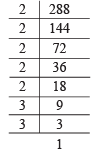i.e. 288 = 2 * 2 * 2 * 2 * 2 * 3 * 3

Grouping the factors in triples, we get

288 = [2 * 2 * 2] * 2 * 2 * 3 * 3

We observe that if 288 is multiplied by (2 * 3), then its prime factors will exist in triples. Thus, the required smallest number by which 288 be multiplied to make it a perfect cube is (2 * 3), i.e. 6.

Question 4: Find the cube of 4/5.

Solution: We have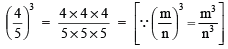= 64/25

Thus,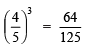Question 5: Show that 0.001728 is the cube of a rational number. Find that rational number whose cube is 0.0017288.

Solution: We have

0.001728  =  1728/1000000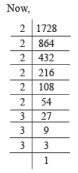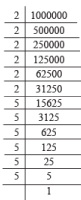∴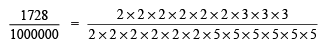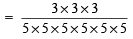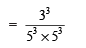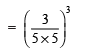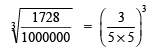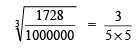⇒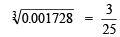Thus, 0.001728 is the cube of  3/25.

Question 6: The volume of a cubical box is 64 cm3. What is its side?

Solution: Let ‘x’ be the side of the cube.

∴  x3 = 64

or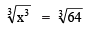⇒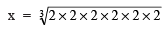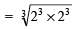= 2 x 2 = 4

Question 7: If the surface area of a cube is 486 cm2. Find its volume.

Solution: A cube has 6 equal surfaces.

Let side of the cube be x.

∴ Area of a surface = x2

∴ Area of the given cube = 6x2

∴ 6x2 = 486

⇒ x2 = 486/6 =  81

⇒ x= √81 = 9

∴ x3 = 9 * 9 * 9 = 729

Thus, the volume of the given cube = 729 cm3.

,

,

,

,

,

,

,

,

,

,

,

,

,

,

,

,

,

,

,

,

,

;